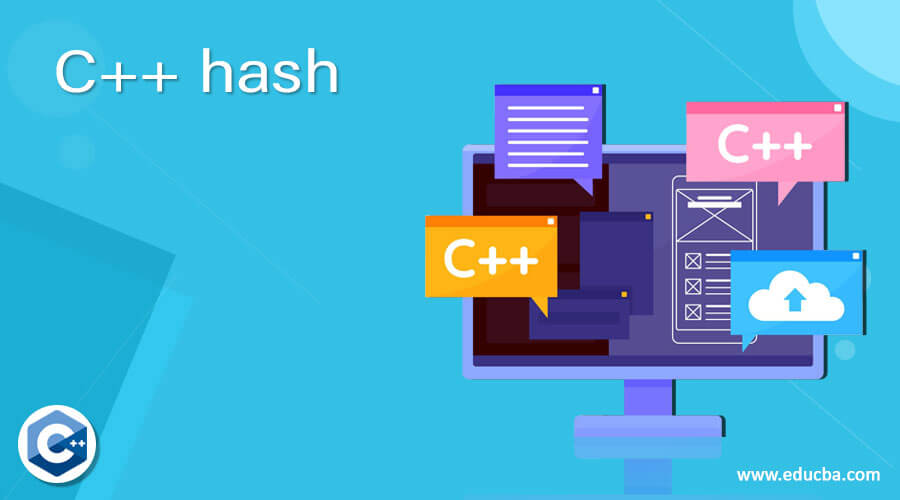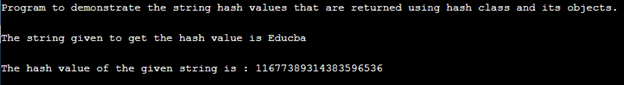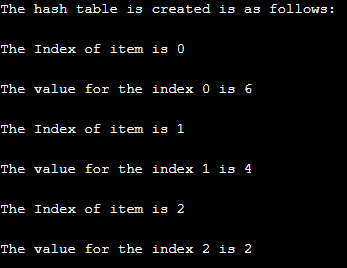# C++哈希## C++哈希简介

### 哈希函数在C++中的工作原理及实例

`template <class key> struct hash;`

`hash<class template> obj_name ;`

`#include <iostream> #include <string> using namespace std; void strhashing() { string h1 = "Educba"; cout <<"The string given to get the hash value is "<< h1 <<"\n"<<endl; hash<string> hash_obj; cout << "The hash value of the given string is : " << hash_obj(h1)<< endl; } int main() { cout<<"Program to demonstrate the string hash values that are returned using hash class and its objects."<<"\n"<<endl; strhashing(); }``#include <iostream> #include <list> using namespace std; class hash_table{`

`list<int> *tbl; int all_ele; int fetch_hash(int k){ return k % all_ele; }`

public:

`hash_table(int a){ all_ele = a; tbl = new list<int>[all_ele]; } void inst_ele(int k){ tbl[fetch_hash(k)].push_back(k); } void disp(){ for(int i = 0; i < all_ele; i++){ cout << "The Index of item is " << i << "\n " <<endl; for(int j : tbl[i]) cout <<"The value for the index "<<i << " is " << j << endl; cout << endl; } } }; int main() { hash_table kh(3); int a[] = {2, 4, 6}; for(int i = 0; i < 3; i++) kh.inst_ele(a[i]); cout << "The hash table is created is as follows: " << "\n"<< endl; kh.disp(); return 0; }`### 推荐文章

The postC++ hashappeared first onEDUCBA.